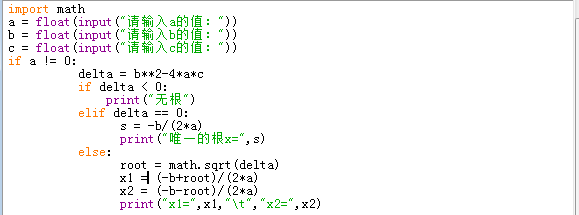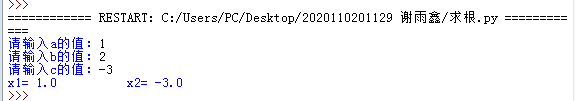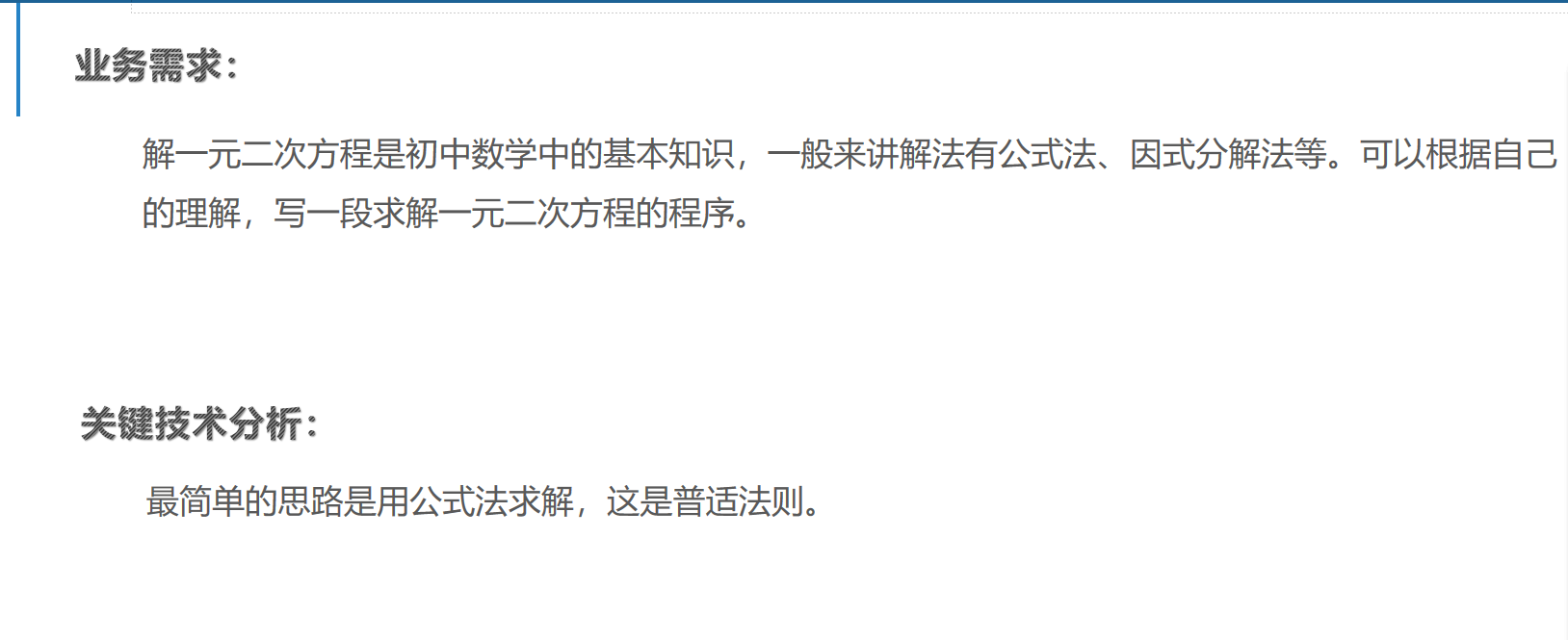• python求解一元二次方程
万次阅读 多人点赞
2020-11-23 13:28:55

我也不知道讲什么，你先想想你解数学题的时候，解方程的数学公式是什么？知道公式再直接转换为代码就ok.有问题留言，我不喜欢多里巴嗦。

import math
print("----计算一元二次方程的根----")

a = float(input("请输入a的值："))
b = float(input("请输入b的值："))
c = float(input("请输入c的值："))
d=b**2-4*a*c
if (d<0):
print("无解")
else:
e = math.sqrt(d)
x1=((-b+e)/(2*a))#调用math模块中sqrt开平方函数
x2=((-b-e)/(2*a))
print("x1=",x1,"\t","x2=",x2)



我是川川，麻烦点个赞，加个关。
QQ：2835809579python
更多相关内容
• 这是一个简单的求解一元二次方程组的python代码，作为新手的第一个完整的代码，还是有很多不足之处python
• 主要介绍了Python实现求解一元二次方程的方法,涉及Python基于math包进行数值运算相关操作技巧,需要的朋友可以参考下
• 首先如图所示敲出下列代码： 即如下 import math a = float(input("请输入a的值：")) b = float(input("请输入b的值：")) c = float(input("请输入c的值："))if a !... delta = b**2-4*a*c ... s ...

首先如图所示敲出下列代码：即如下

import math
a = float(input("请输入a的值："))
b = float(input("请输入b的值："))
c = float(input("请输入c的值："))
if a != 0:
delta = b**2-4*a*c
if delta < 0:
print("无根")
elif delta == 0:
s = -b/(2*a)
print("唯一的根x=",s)
else:
root = math.sqrt(delta)
x1 = (-b+root)/(2*a)
x2 = (-b-root)/(2*a)
print("x1=",x1,"\t","x2=",x2)

输入3个数测试，得到如图所示，即为成功搬走，不客气！

展开全文python 开发语言 后端
• Python求解一元二次方程问题【简单易懂，注释超全，代码可以直接运行】 业务需求: 解一元二次方程是初中数学中的基本知识，- -般来讲解法有公式法、因式分解法等。可以根据自己 的理解，写- -段求解一元二次方程的...

## Python求解一元二次方程问题【简单易懂，注释超全，代码可以直接运行】

业务需求:
解一元二次方程是初中数学中的基本知识，- -般来讲解法有公式法、因式分解法等。可以根据自己
的理解，写- -段求解一元二次方程的程序。
关键技术分析:
最简单的思路是用公式法求解，这是普适法则。这道题利用公式法来解决是非常合适的，首先判断一元二次方程的基本知识，Δ = b² - 4ac的值决定了方程解的个数。然后利用求根公式这道题就结束啦。（-b+根号Δ）/2a,（-b-根号Δ）/2a,就是方程的两个根，求解出来结果即可。

import math

def func(a,b,c):
if (b*b - 4*a*c) < 0:
print("此方程无解！")
elif (b*b - 4*a*c) == 0:
print('此方程有一个解！')
return (0-b+math.sqrt(b*b - 4*a*c))/2*a
else:
print('此方程有两个解！')
return (0 - b + math.sqrt(b*b - 4*a*c))/2*a,(0 - b - math.sqrt(b*b - 4*a*c))/2*a

if __name__ == '__main__':
a = eval(input("请输入二次项的系数："))
b = eval(input("请输入一次项的系数："))
c = eval(input("请输入常数项："))
list = func(a,b,c)
print(list)


点个👍吧，秋梨膏！

展开全文python 算法
• python学习 1

#python一元二次方程求解

# bglei@nuaa.edu.cn 2022年5月11日
import math
z=input('输入一元二次方程系数：').split()
a,b,c=float(z),float(z),float(z)
if b**2==4*a*c:
x= (-b + math.sqrt(b*b-4*a*c))/(2*a)
print('x1=x2=%.5f' %x)
elif b**2>4*a*c:
print('x1=%.5f;x2=%.5f' %((-b + math.sqrt(b*b-4*a*c))/(2*a),(-b - math.sqrt(b*b-4*a*c))/(2*a)))
else:

re= -b / (2*a)
if abs(re-0)<0.000001:
re=0.00000
im=math.sqrt(4*a*c-b*b) / (2*a)
print('x1=%.5f+%.5fi;x2=%.5f-%.5fi' %(re,im,re,im))


运行示例：

输入一元二次方程系数：1 3 4
x1=-1.50000+1.32288i;x2=-1.50000-1.32288i

展开全文python 机器学习 开发语言
• 最近在做笔试题的时候遇到的一道题，挺有意思的贴出来和大佬讨论一下（常规的做法，...考虑到给定的方程需要化简，因此第一步要化简等号左右两端的方程，并获取系数，并存放在字典中，移到等式一端，之后合并字典则6x-python 字符串
• import math def quadratic(a, b, c): if not isinstance(a + b + c, (int, float)): # 验证输入的三个参数是不是数字 raise TypeError("bad operand type") if (b ** 2 - 4 * a * c) <...python
•python pycharm django
• 题目：求一元二次方程ax*x+b*x+c=0的解 从键盘输入a,b,c的值，分多种情况输出解。 a等于0，b也等于0时，输出“方程无解”； a等于0，b不等于0时，输出“方程有1个解，x= ？”；？表示方程的解 a不等于0时，计算...python 开发语言
•python 开发语言
•python
• 1 引入math包 2 定义返回的对象 3 判断b*b-4ac的大小 4 计算代码如下import math class Result: result1 = 0 result2 = 0 def __init__(self, r1, r2): self.result1 = r1 self.result2 = r2 def __returpython 一元二次方程组
• 如何循环python解一元二次方程12345678910111213141516171819202122import numpy as npdef solve_quad(a,b,c):if a == 0:print('您输入的不是方程!')else:delta = b*b-4*a*cx = -b/(2*a)if delta == 0:print('方程有...
• 调用 math.sqrt() 函数一个数的平方根； 使用 def 自定义一个函数时，函数返回值使用 return 返回； 函数体内部的语句在执行时，一旦执行到 return 时，函数就执行完毕，并将结果返回； 函数的返回值可以是多个...
• 本文实例讲述了Python编程实现数学运算求一元二次方程的实根算法。分享给大家供大家参考，具体如下： 问题： 请定义一个函数quadratic(a, b, c)，接收3个参数，返回一元二次方程：ax² + bx + c = 0的两个解。 实现...
• #一元二次方程 a,b,c=float(input('a')),float(input('b')),float(input('c')) m=(b**2-4*a*c)**0.5 if m>=0: print((m-b)/2/a,(-m-b)/2/a) 无实数根时报错 ValueError: negative number cannot be raised to a...
• 利用公式x1 = (-b + sqrt(bb-4ac))/(2a), x2 = (-b - sqrt(bb-4ac))/(2a)求一元二次方程ax2+ bx + c =0的根，其中a不等于0。 输入: 输入一行，包含三个浮点数a, b, c（它们之间以一个空格分开），分别表示方程ax2 + ...
• python求一元次方程 # 方程求解 import sympy as sy import sympy import sympy as sp # 导入sympy包 import math a=math.pi #-----------------方法一---------------------- x = sp.Symbol('x') # 定义符号变量...python
• 背景：如何使用python求解多元多次方程组或者非线性方程组。 原创内容，转载注明出处！请勿用于商业用途！ （上篇用python拟合2019nCov感染人数的文章被不少博主转载了，发的比较早，不少博主在文章基础上添加新内容...
• 一元二次方程：ax²+ bx + c = 0 根公式：x =（ - b +√（b²-4ac））/ 2a  判别式：称称b²-4AC def my_math(a, b , c): #首先判断输入的参数为int、float类型，如果不是则输出自定义异常。 if not ...
• 计算机语言运用--数值计算9-方程的计算机处理92-4_Fortran数值计算方程的计算机处理这里说的方程，是以实数域为计算范围。这里说的方程：是含有未知数的等式。可以是a+5=8，或X+A=B，或ax2+bx+c=0，或ax+by=c与dx-ey...
•python 开发语言 后端
• python求解一元二次方程 工具：spyder3 环境：Windows10、python3.6 目的：对基本数据类型、输入输出进行基础复习 #coding=utf8 import math a=int(input("请输入a：")); b=int(input("请输入b：")); c=int(input...python 编程语言...